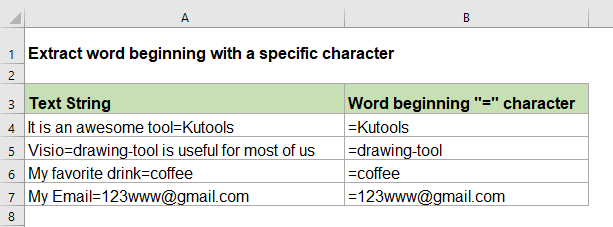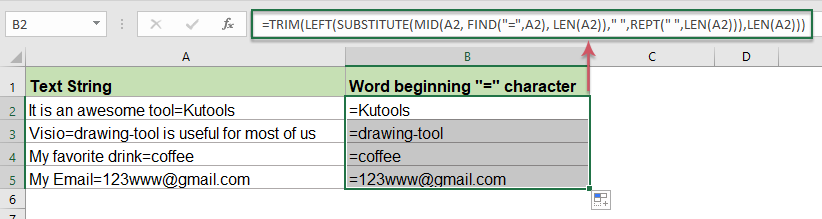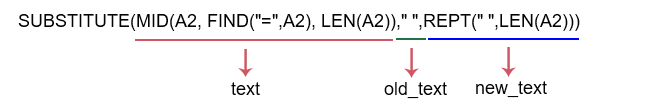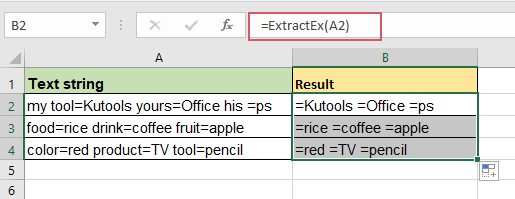## Extract word beginning with a specific character in Excel

For example, you may need to extract the word which begins with a specific character “=” for each cell as below screenshot shown, how could you solve this task quickly and easily in Excel?#### Extract word beginning with a specific character in Excel

To extract the words that begin with a specific character or text from each cell, you can create a formula with a combination of TRIM, LEFT, SUBSTITUTE, MID, FIND, LEN and REPT functions, the generic syntax is:

=TRIM(LEFT(SUBSTITUTE(MID(text, FIND(char, text), LEN(text))," ",REPT(" ",LEN(text))),LEN(text)))
• text: The text string or cell value that you want to extract word from.
• char: The character or text that you want to extract word begins.

1. Please copy or enter the following formula into a blank cell:

=TRIM(LEFT(SUBSTITUTE(MID(A2, FIND("=",A2), LEN(A2))," ",REPT(" ",LEN(A2))),LEN(A2)))

2. Then, drag the fill handle down to apply the formula to the cells that you want to use, and all the words begin with the specific “=” character have been extracted at once, see screenshot:##### Explanation of the formula:

1. MID(A2, FIND("=",A2), LEN(A2):

• LEN(A2): This LEN function returns the number of the characters in cell A2. This part is recognized as the num_char argument in the MID function.
• FIND("=",A2): This FIND function is used to get the position of the first specific character “=” in cell A2. This part is recognized as the start_num argument in the MID function.
• MID(A2, FIND("=",A2), LEN(A2): This MID function is used to extract a substring from cell A2 at the position which returned by the FIND function and specific length returned by the LEN function.

2. SUBSTITUTE(MID(A2, FIND("=",A2), LEN(A2))," ",REPT(" ",LEN(A2))):

• REPT(" ",LEN(A2): The REPT function repeats empty string of cell A2 a specific number of times returned by the LEN function.
• SUBSTITUTE(): This SUBSTITUTE function will replace all empty string with another new text-multiple spaces returned by the REPT function from a text string returned by the MID function.
•• MID(A2, FIND("=",A2), LEN(A2): This MID function is used to extract a substring from cell A2 at the position which returned by the FIND function and specific length returned by the LEN function.

3. LEFT(SUBSTITUTE(MID(A2, FIND("=",A2), LEN(A2))," ",REPT(" ",LEN(A2))),LEN(A2)):This LEFT function is used to extract the specific number of characters returned by the LEN function from left side of the text string returned by the SUBSTITUTE function.

4. TRIM():The TRIM function remove all extra spaces from text string returned by the LEFT function.

Notes:

1. In the above formula, you can change the “=” character to any other character or text you need.

2. If there are multiple words which begin the specific character, only the first word will be extracted.

#### Relative functions used:

• REPT:
• The REPT function is used to repeat the characters a specified number of times.
• SUBSTITUTE:
• The SUBSTITUTE function replaces text or characters within a text string with another text or characters.
• TRIM:
• The TRIM function removes all extra spaces from text string and only keeps single spaces between words.
• MID:
• The MID function returns the specific characters from the middle of text string.
• LEN:
• The LEN function returns the number of characters in a text string.
• REPT:
• The REPT function is used to repeat the characters a specified number of times.
• FIND:
• The FIND function is used to find a string within another string, and returns the starting position of the string inside another one.

#### More articles:

• Extract Word Which Containing Specific Text In Excel
• Sometimes, you may want to extract the word from a cell that contains a specific character or text, such as following screenshot shown, to extract all words that containing the “=” character. How could you solve this task in Excel?
• Extract Text Between Parentheses From Text String
• If there is part of the text surrounded with the parentheses within the text string, now, you need to extract all the text strings between the parentheses as following screenshot shown. How could you solve this task in Excel quickly and easily?
• Extract Multiple Lines From A Cell
• If you have a list of text strings which are separated by line breaks (that occurs by pressing Alt + Enter keys when entering the text), and now, you want to extract these lines of text into multiple cells as below screenshot shown. How could you solve it with a formula in Excel?

### The Best Office Productivity Tools

#### Kutools for Excel - Helps You To Stand Out From Crowd

 Popular Features: Find, Highlight or Identify Duplicates  |  Delete Blank Rows  |  Combine Columns or Cells without Losing Data  |  Round without Formula ... Super VLookup: Multiple Criteria  |  Multiple Value  |  Across Multi-Sheets  |  Fuzzy Lookup... Adv. Drop-down List: Easy Drop Down List  |  Dependent Drop Down List  |  Multi-select Drop Down List... Column Manager: Add a Specific Number of Columns  |  Move Columns  |  Toggle Visibility Status of Hidden Columns  |  Compare Columns to Select Same & Different Cells ... Featured Features: Grid Focus  |  Design View  |  Big Formula Bar  |  Workbook & Sheet Manager | Resource Library (Auto Text)  |  Date Picker  |  Combine Worksheets  |  Encrypt/Decrypt Cells  |  Send Emails by List  |  Super Filter  |  Special Filter (filter bold/italic/strikethrough...) ... Top 15 Toolsets:  12 Text Tools (Add Text, Remove Characters ...)  |  50+ Chart Types (Gantt Chart ...)  |  40+ Practical Formulas (Calculate age based on birthday ...)  |  19 Insertion Tools (Insert QR Code, Insert Picture from Path ...)  |  12 Conversion Tools (Numbers to Words, Currency Conversion ...)  |  7 Merge & Split Tools (Advanced Combine Rows, Split Excel Cells ...)  |  ... and more

Kutools for Excel Boasts Over 300 Features, Ensuring That What You Need is Just A Click Away...#### Office Tab - Enable Tabbed Reading and Editing in Microsoft Office (include Excel)

• One second to switch between dozens of open documents!
• Reduce hundreds of mouse clicks for you every day, say goodbye to mouse hand.
• Increases your productivity by 50% when viewing and editing multiple documents.
• Brings Efficient Tabs to Office (include Excel), Just Like Chrome, Edge and Firefox.No ratings yet. Be the first to rate!
This comment was minimized by the moderator on the site
This formula works for a single match only. What if there are multiple matches?
This comment was minimized by the moderator on the site
Hello, Mdhdy,
If you need to extract all matches from a cell, the following User Defined Function may help you:
Note: In the code, please change the "=" character from this sctipt .Pattern = "=\S+" to any other character you need.
``````Function ExtractEx(Target As Range) As String
ExtractEx = ""
If Target.Count > 1 Then Exit Function
On Error Resume Next

Dim xRetList As Object
Dim xRegEx As Object
Dim I As Long
Dim xRet As String
Application.Volatile
Set xRegEx = CreateObject("VBSCRIPT.REGEXP")
With xRegEx
.Pattern = "=\S+"
.Global = True
.MultiLine = True
.IgnoreCase = True
End With
Set xRetList = xRegEx.Execute(Target.Formula)

If xRetList.Count > 0 Then
For I = 0 To xRetList.Count - 1
xRet = xRet & xRetList.Item(I) & " "
Next
ExtractEx = xRet
Else
ExtractEx = ""
End If

End Function
``````

After pasting the code, please apply this formula：=ExtractEx(A2), see the below screenshot:There are no comments posted here yet